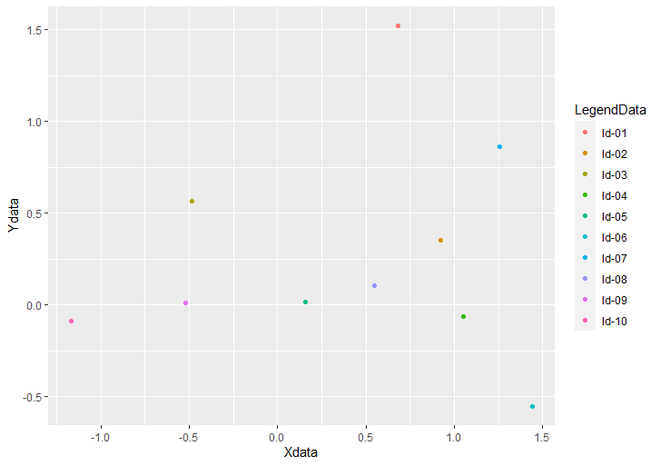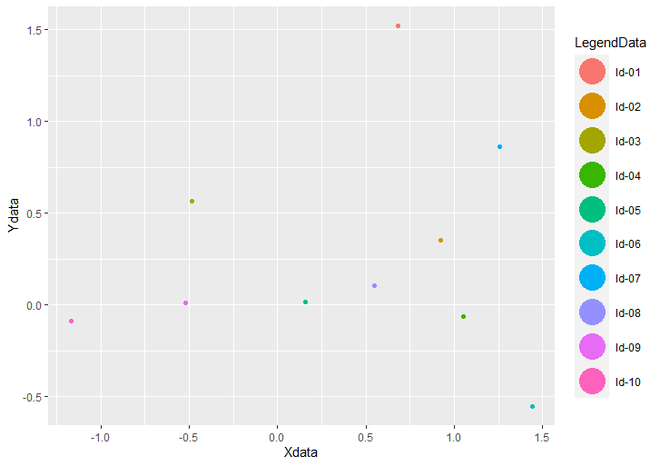Related Articles

# Control Size of ggplot2 Legend Items in R

• Last Updated : 30 May, 2021

In this article, we will see how to control the size of ggplot2 Legend Items in R Programming Language. To create an R plot, we use ggplot() function and for making a scatter plot geom_point() function is added to ggplot() function.

Let us first create a regular plot without any modifications so that the difference is apparent.

Example:

## R

 `# Load Package``library``(``"ggplot2"``)`` ` `# Create a DataFrame ``data <- ``data.frame``(Xdata = ``rnorm``(10),                        ``                   ``Ydata = ``rnorm``(10),``                   ``LegendData = ``c``(``"ld-01"``, ``"ld-02"``, ``"ld-03"``,``                                  ``"ld-04"``, ``"ld-05"``, ``"ld-06"``,``                                  ``"ld-07"``, ``"ld-08"``, ``"ld-09"``, ``                                  ``"ld-10"``))`` ` `# Create a Scatter Plot``ggplot``(data, ``aes``(Xdata, Ydata, color = LegendData)) +   ``  ``geom_point``()`

Output:Scatter Plot with Legend

To change the Size of Legend, we have to add guides() and guide_legend() functions to the geom_point() function. Inside guides() function, we take parameter color, which calls guide_legend() guide function as value. Inside guide_legend() function, we take an argument called override.aes, which has the list specifying aesthetic parameters of legend keys. Inside this list, we specify the size of legend.

Syntax : guides(…)

Parameter :

• … : either a string or call to a guide function. here we call guide_legend() guide function.

Return : each scale can be set scale-by-scale

Syntax : guide_legend(override.aes = list())

Parameter :

• override.aes : A list specifying aesthetic parameters of legend key. Inside this list, we specify the size of legend to size object.

Return : Legend Guides for various scales

Example:

## R

 `# Load Package``library``(``"ggplot2"``)`` ` `# Create a DataFrame ``data <- ``data.frame``(Xdata = ``rnorm``(10),                        ``                   ``Ydata = ``rnorm``(10),``                   ``LegendData = ``c``(``"ld-01"``, ``"ld-02"``, ``"ld-03"``,``                                  ``"ld-04"``, ``"ld-05"``, ``"ld-06"``,``                                  ``"ld-07"``, ``"ld-08"``, ``"ld-09"``, ``                                  ``"ld-10"``))`` ` `# Create a Scatter Plot and change``# the size of legend``ggplot``(data, ``aes``(Xdata, Ydata, color = LegendData)) +   ``  ``geom_point``()+``  ``guides``(color = ``guide_legend``(override.aes = ``list``(size = 10)))`

Output:Scatterplot with change size of legend

My Personal Notes arrow_drop_up